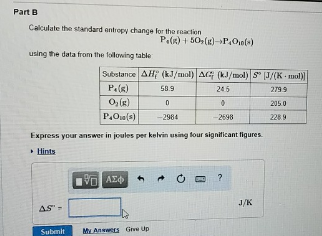# Problem: Part BCalculate the standard entropy change for the reaction P4 (g) + 5O2 (g) → P4O10 (s) using the data from the following table: Express your answer in joules per kelvin using four significant figures.

###### FREE Expert Solution
89% (301 ratings)###### Problem Details

Part B

Calculate the standard entropy change for the reaction

P4 (g) + 5O2 (g) → P4O10 (s)

using the data from the following table: